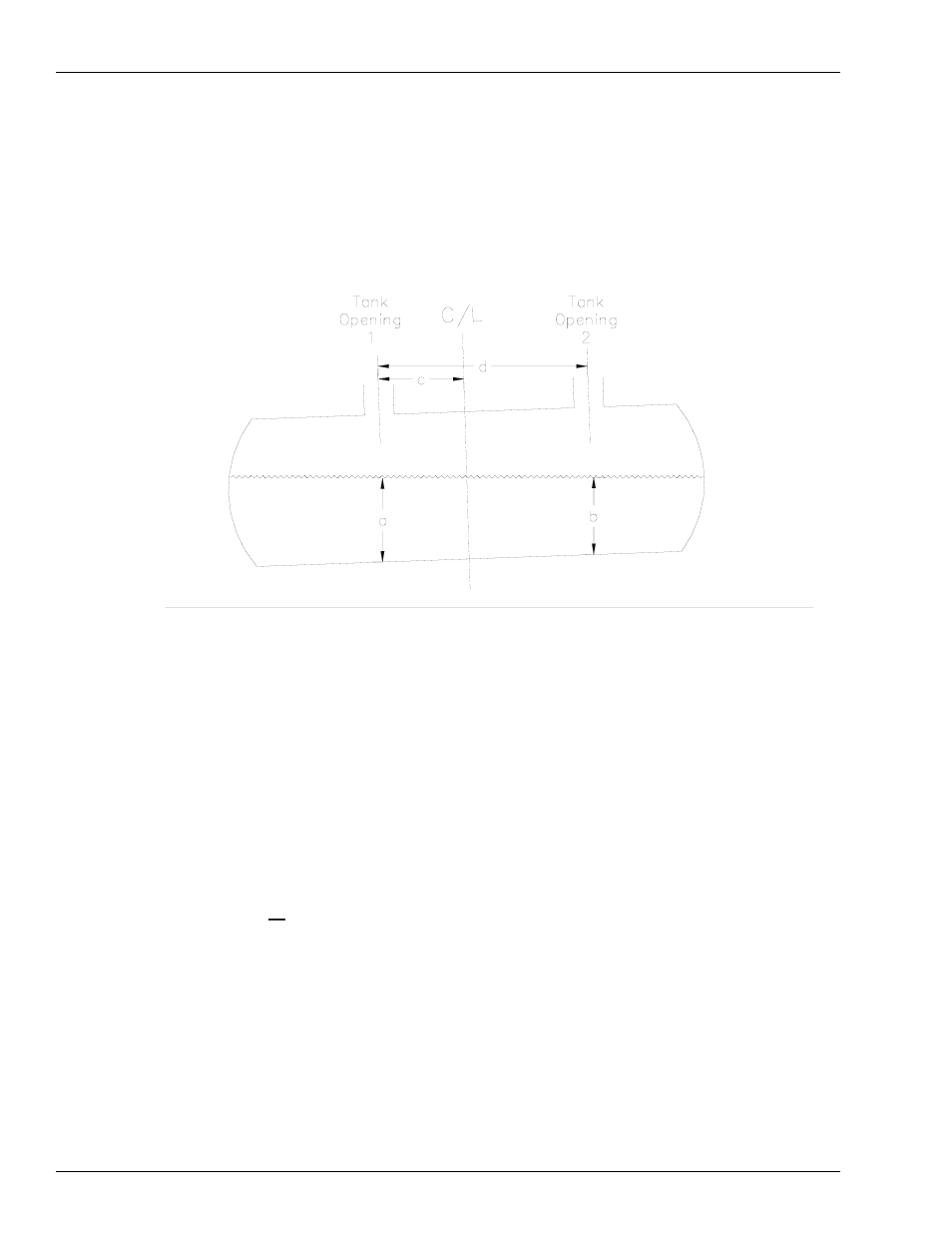# Calculating your tank tilt offset, 6 calculate the offset using the following formula, Calculating your tank tilt offset -18 – Gasboy PC CFN site controller User Manual

## Page 22PC/CFN Basics

Page 18

MDE-4489 Gasboy® PC/CFN User’s Guide·July 2005

Due to installation or ground settling, the underground storage tank may be tilted. This could
result in inaccurate inventory volume readings. You must measure for tank tilt so that an
Offset can be entered for each tank on the Tank Information Form. Use the following figure
and formula to determine your tank's offset (tilt) factor.

1

Using a dipstick, measure the product level at Tank Opening 1 (a)

2

Using a dipstick, measure the product level at Tank Opening 2 (b).

3

If a=b, the Offset is 0 and no further calculations are needed.

4

Measure the distance between Tank Opening 1 and the tank center line (c).

5

Measure the distance between Tank Opening 1 and Tank Opening 2 (d).

6

Calculate the offset using the following formula:

OFFSET = c (a-b)
d